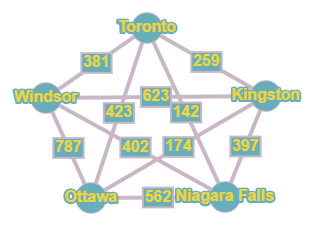# Travel A tourist is staying in Toronto, Canada, and would like to visit four other Canadian cities by train. The visitor wants to go from one city to the next and return to Toronto whileminimizing the total travel distance. The distances between cities. in kilometers, are given in the following table. Represent the distances between the cities using a weighted graph. Use the greedy algorithm to plan a route for the tourist.### Mathematical Excursions (MindTap C...

4th Edition
Richard N. Aufmann + 3 others
Publisher: Cengage Learning
ISBN: 9781305965584

#### Solutions

Chapter
Section### Mathematical Excursions (MindTap C...

4th Edition
Richard N. Aufmann + 3 others
Publisher: Cengage Learning
ISBN: 9781305965584
Chapter 5.2, Problem 20ES
Textbook Problem
61 views

## Travel A tourist is staying in Toronto, Canada, and would like to visit four other Canadian cities by train. The visitor wants to go from one city to the next and return to Toronto whileminimizing the total travel distance. The distances between cities. in kilometers, are given in the following table. Represent the distances between the cities using a weighted graph. Use the greedy algorithm to plan a route for the tourist.To determine

To represent the distances between the cities by a weighted graph and to plan a route for the tourist using the greedy algorithm.

### Explanation of Solution

Given information:

Given, a tourist is staying in Toronto, Canada, and would like to visit four other Canadian cities by train.

The visitor wants to go from one city to the next and return to Toronto while minimizing the total travel distance.

The distances between cities, in kilometers, are given in the following table:

Calculation:

Given, there are five cities out of which the tourist stays in Toronto, Canada.

Hence five vertices corresponding to the five cities are marked with respective names.

And then making the complete graph by marking every possible edge with every edge marked with the weight represented in the table between the cities.

The distance between Toronto and Kingston is 259. Hence the edge drawn between them has a weight of 259

Similarly marking all the edges with respective weights, the graph is given as:

To use the greedy algorithm, the graph must be Hamiltonian.

(Since, the tourist needs to visit each city only once)

A path in a Hamiltonian graph is said to be a Hamiltonian Circuit if it begins and ends at the same vertex and passes through each vertex of a graph exactly once.

The greedy algorithm states to travel along a connected edge that has the smallest weight that is not yet visited. This process continues till all the vertices are visited and returned to the starting vertex.

Here, the vertex representing Toronto is selected.

Vertex representing Toronto is of degree 4 and the weights of the connected edges are 259, 142, 423, 381

Hence, as per the Greedy Algorithm the edge with weight 142 is selected i...

### Still sussing out bartleby?

Check out a sample textbook solution.

See a sample solution

#### The Solution to Your Study Problems

Bartleby provides explanations to thousands of textbook problems written by our experts, many with advanced degrees!

Get Started

Find more solutions based on key concepts
In Problems 76-82, perform the indicated operations and simplify. x+2x2xx2+4x22x+1+1

Mathematical Applications for the Management, Life, and Social Sciences

Find the maximum revenue R (in dollars) given that 12(2R 320) 4(3R + 240)

Applied Calculus for the Managerial, Life, and Social Sciences: A Brief Approach

Factor: 20y310y2+5y

Elementary Technical Mathematics

Determine whether the series converges or diverges. 9. k=1lnkk

Single Variable Calculus: Early Transcendentals

Prove the commutative law for disjunction, pqqp.

Finite Mathematics for the Managerial, Life, and Social Sciences

Using for |x| < 1 and differentiation, find a power series for .

Study Guide for Stewart's Single Variable Calculus: Early Transcendentals, 8th

Special Rounding Instructions For this exercise set, round all regression parameters to three decimal places, b...

Functions and Change: A Modeling Approach to College Algebra (MindTap Course List)